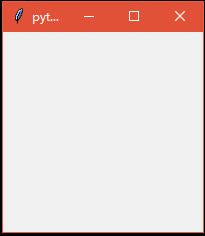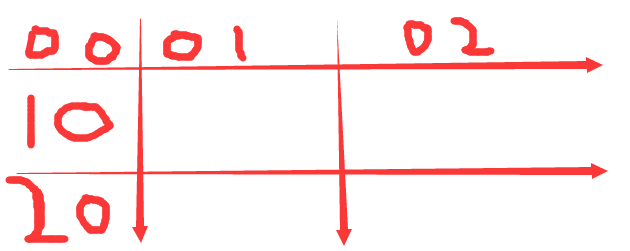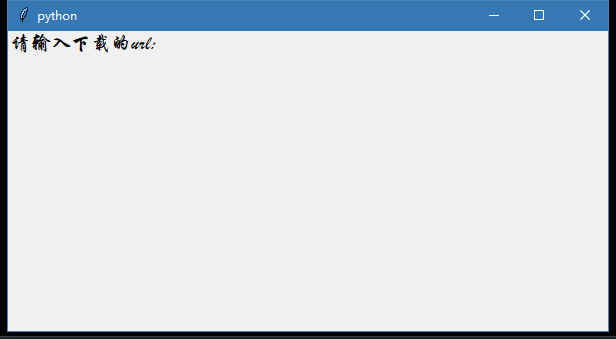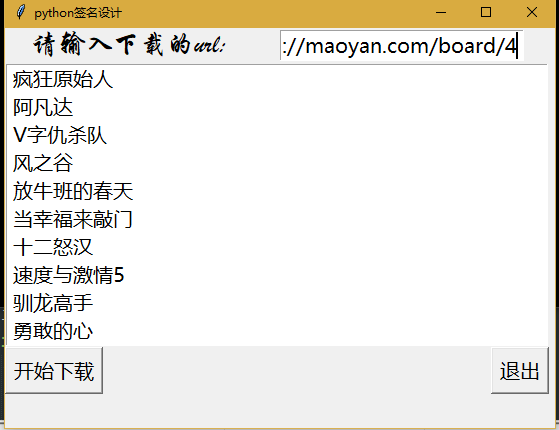# python3爬虫实战（五）：为爬虫添加GUI图像界面

## 一、前言

前面我们写的爬虫只能运行在具有python环境的电脑上，若是把源代码发给别人，很大可能性是运行不了的，所以我们本节的目的是为爬虫创建GUI界面，并打包成exe可执行文件。

## 二、学习知识点

tkinter：tkinter是python内置的模块，不用安装，可直接导入

tkinter的学习是参考b站的一个python gui界面设计的视频 Python教程：https://www.bilibili.com/video/av26214247?from=search&seid=10819799360989272623

from tkinter import *

def main():

#创建空白窗口,作为主载体
root = Tk()
root.title('python')
#显示窗口
mainloop()

main()root.geometry('600x300+398+279')

#标签控件，窗口中放置文本组件
Label(root,text='请输入下载的url:',font=("华文行楷",15),fg='black').grid()#Entry是可输入文本框
url_input=Entry(root,font=("微软雅黑",15),width=50)
url_input.grid(row=0,column=1)

grid这次定位在0行1列，与上一个标签控件在同一行

#列表控件
text=Listbox(root,font=('微软雅黑',15),width=45,height=10)
#columnspan 组件所跨越的列数
text.grid(row=1,columnspan=2)

columnspan表示合并两个列

button =Button(root,text='开始下载',font=("微软雅黑",15),command=Crawl_content).grid(row=2,column=0,sticky=W)
button =Button(root,text='退出',font=("微软雅黑",15),command=root.quit).grid(row=2,column=1,sticky=E)

from tkinter import *

def main():
global url_input,text
#创建空白窗口,作为主载体
root = Tk()
root.title('测试——猫眼电影')
#窗口的大小，后面的加号是窗口在整个屏幕的位置
root.geometry('550x400+398+279')
#标签控件，窗口中放置文本组件
Label(root,text='请输入下载的url:',font=("华文行楷",20),fg='black').grid()
#定位 pack包 place位置 grid是网格式的布局

#Entry是可输入文本框
url_input=Entry(root,font=("微软雅黑",15))
url_input.grid(row=0,column=1)
#列表控件
text=Listbox(root,font=('微软雅黑',15),width=45,height=10)
#columnspan 组件所跨越的列数
text.grid(row=1,columnspan=2)
#设置按钮 sticky对齐方式，N S W E
button =Button(root,text='开始下载',font=("微软雅黑",15)).grid(row=2,column=0,sticky=W)
button =Button(root,text='退出',font=("微软雅黑",15),command=root.quit).grid(row=2,column=1,sticky=E)
#使得窗口一直存在
mainloop()

main()

from tkinter import *
import requests
import os
from bs4 import BeautifulSoup
from pyquery import PyQuery as pq
import csv
os.chdir('E:\爬虫数据')

def Crawl_content():
url=url_input.get()
headers={'user-agent':'user-agent'}
file=csv.writer(open('猫眼电影.csv','w'))
for i in range(0,100,10):
new_url=url+'?offset=%d'%i
print('在解析网址中：',new_url)
req=requests.get(url=new_url,headers=headers)
html=req.text
doc=pq(html)
items=doc('dl.board-wrapper dd').items()
for each in items:
title=each.find('a').text()
#添加数据
text.insert(END,title)
#文本框向下滚动
text.see(END)
#更新
text.update()
print('已抓取完毕')

def main():
global url_input,text
#创建空白窗口,作为主载体
root = Tk()
root.title('测试——猫眼电影')
#窗口的大小，后面的加号是窗口在整个屏幕的位置
root.geometry('550x400+398+279')
#标签控件，窗口中放置文本组件
Label(root,text='请输入下载的url:',font=("华文行楷",20),fg='black').grid()
#定位 pack包 place位置 grid是网格式的布局

#Entry是可输入文本框
url_input=Entry(root,font=("微软雅黑",15))
url_input.grid(row=0,column=1)
Label(root,text='猫眼电影url: http://maoyan.com/board/4',font=("微软雅黑",10),fg='black').grid(row=1)
#列表控件
text=Listbox(root,font=('微软雅黑',15),width=45,height=10)
#columnspan 组件所跨越的列数
text.grid(row=2,columnspan=2)
#设置按钮 sticky对齐方式，N S W E
button =Button(root,text='开始下载',font=("微软雅黑",15),command=Crawl_content).grid(row=3,column=0,sticky=W)
button =Button(root,text='退出',font=("微软雅黑",15),command=root.quit).grid(row=3,column=1,sticky=E)
#使得窗口一直存在
mainloop()

main()
# http://maoyan.com/board/4


url_input把它设置为全局变量，在爬虫抓取的时候可以通过get()获得我们输入的url，同样为了让抓取的内容显示在我们的列表控件中，我们需要把text这个列表控件也设置为全局变量，然后在爬虫中用text.insert()插入数据。pip install pyinstaller